# −1 (number) facts for kids

Kids Encyclopedia Facts
(Redirected from −1)

In mathematics, −1 is the additive inverse of 1. That is, if it is added to 1, the result is 0. It is the negative integer greater than negative two (−2) and less than 0.

Negative one has some similar properties as positive one. But some are different.

Negative one is related to Euler's identity. This is because the identity states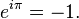$e^{i \pi} = -1.\!$

In computer science, −1 is a common initial value for integers. It is also used to show that a variable has no useful information.

## Algebraic properties

Multiplying a number by −1 is the same as changing the sign on the number. This can be proved using the distributive law and the axiom at 1 is the multiplicative identity, that is, a number multiplied by 1 is the number itself. So, for x real, we have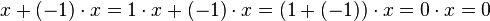$x+(-1)\cdot x=1\cdot x+(-1)\cdot x=(1+(-1))\cdot x=0 \cdot x=0$

where we used the fact that 0 multiplied by any real number x equals 0, shown by cancellation from the equation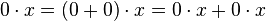$0\cdot x=(0+0)\cdot x=0\cdot x+0\cdot x \,$

In other words,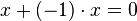$x+(-1)\cdot x=0 \,$

so (−1) · x or −x is the arithmetic inverse of x.

### Square of −1

The square of −1, i.e. −1 multiplied by −1, equals 1. So, a square of negative real numbers is positive.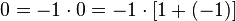$0 =-1\cdot 0 =-1\cdot [1+(-1)]$

The first equality follows from the above result. The second follows from the definition of −1 as additive inverse of 1, that is, when added to 1, it gives 0. Now, using the distributive law, we see that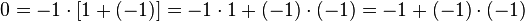$0 =-1\cdot [1+(-1)]=-1\cdot1+(-1)\cdot(-1)=-1+(-1)\cdot(-1)$

The second equality follows from the fact that 1 is a multiplicative identity, that is :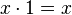$x\cdot 1=x \,$. But now adding 1 to both sides of this last equation means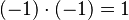$(-1) \cdot (-1) = 1$

The above arguments hold in any ring. Ring is a concept of abstract algebra generalizing integers and real numbers.

### Square roots of −1

The complex number i satisfies i2 = −1. So it is a square root of −1. The only other complex number x for which the equation x2 = −1 holds is −i. In the algebra of quaternions, which has the complex plane, the equation x2 = −1 has an infinity of solutions.

## Exponentiation to negative integers

A non-zero real number can have a negative number as its power. We define that x−1 = 1/x. This means a number raised to a power of −1 is equal to the reciprocal of that number. The exponential law xaxb = x(a + b) for a,b non-zero real numbers is true even if a or b is negative.

## Computer representation

There are many ways that −1 (and negative numbers in general) can be represented in computer systems. The most common is as two's complement of their positive form. In standard binary representation, this can also represent a positive integer.−1 (number) Facts for Kids. Kiddle Encyclopedia.